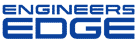Reciprocating Engine Formula Equations 4-Stroke

General Engineering Reviews
Engineering Applications and Design

The following tables define equations for four stroke reciprocating engines.

 Power BHP = PLAN/33,000 P is brake mean effective pressure, in PSI L is piston stroke, in feet A is the area of one piston, in square inches N is the number of power strokes per minute Piston Speed   Cm = .166 x L x N Cm is mean piston speed, in feet per minute L is stroke, in inches N is crankshaft speed, in RPM Brake Mean Effective Pressure (BMEP)   2-Stroke BMEP = (HP x 6500)/(L x RPM) 4-Stroke BMEP = (HP x 13000)/(L x RPM) L = Displacement in Liters   i.e., 80 cc = .08 Liters 1 ci. = 16.39 cc Piston Acceleration   Gmax = ((N2 x L)/2189) x (1 + 1/(2A)) Gmax is maximum piston acceleration, in feet per second squared N is crankshaft speed, in RPM L is stroke, in inches A is the ratio of connecting rod length, between centers, to stroke Piston Stroke Motion S = R cos X + L cos Z S = the distance piston wrist pin is from center of crankshaft R = the radius of the crankshaft wrist pin L = the length of the connecting rod X = the angle of the wrist pin Z = the angle of the connecting rod or sin X = R/L sin Z Piston Travel vs. Crank Rotation   d = ((S/2) + L) - (S/2 cos X) - L sin[cos-1 (S/2L sin X)] S = Stroke (mm) L = Connecting Rod Length (mm) X = Crank Angle Before or After TDC (deg) Note: (L) Rod Length is usually 2 times the (S) Stroke OR For Spreadsheets and some Calculators HT = (r + c) - (r cos (a)) - SQRT(c2 - (r sin (a))2) r = s/2 dtor = PI/180 a = d x dtor HT = The height of piston r = The stroke divided by 2 c = The rod length a = The crank angle in radians d = The crank angle in degrees dtor = Degrees to Radians Exhaust Systems Tuned Length   Lt = (Eo x Vs) / N Lt is the tuned length, in inches Eo is the exhaust-open period, in degrees Vs is wave speed in feet per second (1700 ft/sec at sea level) N is crankshaft speed, in RPM Length of Curved Pipe L = R x .01745 x Z L is length R is radius of the pipe bend Z is the angle of the bend Diffuser Proportions D2 = SQRT( D1^2 x 6.25 ) D2 is the diffuser outlet diameter D1 is the diffuser inlet diameter 6.25 is the outlet/inlet ratio constant Baffle Cones Lr = Le/2 Lr is mean point of the reflection inside the baffle cone Le is the length of the baffle cone Port Open Time   T = ( 60/N ) x ( Z/360 ) or T = Z/( N x 6) T is time, in seconds N is crankshaft speed, in RPM Z is port open duration, in degrees Compression Ratio   CR = ( V1 + V2 ) / V2 CR is compression ratio V1 is cylinder volume at exhaust closing V2 is combustion chamber volume Carburetor Throttle Bore Diameter   D = K x SQRT( C x N ) D is throttle bore diameter, in millimeters K is a constant ( approx. 0.65 to 0.9, derive from existing carburetor bore) C is cylinder displacement, in liters N is RPM at peak power Crankcase Volume   Primary compression ratio = Case Volume @ TDC / Case Volume at BDC or CRp = V1 + V2 / V1 CRp is the primary compression ratio V1 is crankcase volume @ BDC V2 is piston displacement Resonance Effects   F = Vs / 2 * the square root of A / Vc (L + 1/2 the square root of   A Vs is the sonic speed Usually about 1100 ft/sec) A is the cross-sectional area of the inlet L is the inlet pipe length Vc is the flask (crankcase) volume Average Exhaust Temperature   Exhaust gas temperature in Kelvin (k = C + 273.15). This is usually a function of the engine's BMEP.
 MPH Calculate To figure miles per hour, multiply the engine RPM by the Wheel Diameter in inches and divide this by the Gear Ratio times 336 or MPH = RPM * wheel diameter (in inches) / gear ratio * 336 RPM Calculate To figure engine speed (RPM), multiply by the Speed in MPH, by the rear axle gear ratio times 336. Divide this by the tire diameter in inches. or RPM = MPH * gear ratio * 336 / tire diameterMembership Register | LoginHomeEngineering Book StoreEngineering ForumExcel App. DownloadsOnline Books & ManualsEngineering NewsEngineering VideosEngineering CalculatorsEngineering ToolboxGD&T Training Geometric Dimensioning TolerancingDFM DFA TrainingTraining Online EngineeringAdvertising CenterCopyright Notice